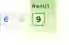VB.NET Random NumbersUse the Random class. Random has a Next Function and is often used as a field.
dot net perls

Random. Z, M, E, L. You could try to decipher the secret order of those letters. But there is none. They are random—the ordering is randomized.Random details. The Random type returns a stream of numbers. It is best used as a field—this improves results when called from multiple methods.

Next method. We create a Random class instance with its constructor. And with Next, we pass up to 2 arguments. Default values are used if we do not specify them as arguments.

Argument 1 This is the minimum value returned by Next. It is inclusive, so can be a possible result.

Argument 2 This is the exclusive (not included) maximum. A max of 10 means the highest result possible is 9.VB.NET program that uses random, Next
Module Module1 Sub Main() Dim r As Random = New Random ' Get random numbers between 5 and 10. ' ... The values 5 through 9 are possible. Console.WriteLine(r.Next(5, 10)) Console.WriteLine(r.Next(5, 10)) Console.WriteLine(r.Next(5, 10)) End Sub End Module
6 9 5

Field. For modules or classes, it is best to store a Random number generator as a field. The Random instance will not repeat itself as much.

Here The program writes three random numbers to the screen. The output will vary each time you run it.

VB.NET program that uses field, module
Module Module1 Sub Main() ' Write three random numbers. F() F() F() End Sub ''' <summary> ''' Write the next random number generated. ''' </summary> Private Sub F() ' Call Next method on the random object instance. Console.WriteLine(_r.Next) End Sub ''' <summary> ''' Store a random number generator. ''' </summary> Private _r As Random = New Random End Module
1284029964 984949494 1322530626

Random bytes. Sometimes we need to generate many random numbers at once. If we need bytes, we can generate random bytes with NextBytes.

Info A loop that generates each byte individually could be used, but NextBytes is clearer and simpler to read.

VB.NET program that generates random bytes with NextBytes
Module Module1 Sub Main() Dim randomGenerator = New Random() ' Array of 10 random bytes. Dim buffer(9) As Byte ' Generate 10 random bytes. randomGenerator.NextBytes(buffer) For Each value In buffer Console.Write("[{0}]", value) Next End Sub End Module


Overloads. How does the Next Function work on the Random type? You can use overloaded versions of the Next Function to constrain the range of the Random number yielded.

Review The first argument is the inclusive lower bound. The second argument is the exclusive upper bound.

However If no arguments are passed, any positive number is returned. If one argument is used, that is the max.

Random array element. We can use the Choose() Function to get an array element in VB.NET. And with the Random Next() Function as an index, we can get a random array element.

Choose

Random lowercase letters. Sometimes in programs we need random lowercase letters. These are just ASCII characters that come in a pseudo-random order.

Random Lowercase Letter

A review. It is important to use the Random type as a field in some situations, to reduce the possibility of repetition. Having fewer Random number generators is also more efficient.

Home
© 2007-2021 sam allen. send bug reports to info@dotnetperls.com.